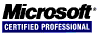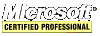# Intel IA-32 Assembly Tutorial

There are five integer addition/subtraction instructions: `INC DEC ADD SUB NEG`.

`INC` and `DEC` increment and decrement by one.

``````	.data
var1 DWORD 1
.code
xor eax,eax     ;set eax to zero
inc eax         ;eax equals one
dec eax         ;eax equals zero again
dec var1        ;var1 equals zero
``````

`ADD` adds two operands. The first operand is the destination and the second operand is the source. One operand can be a memory variable or both can be registers.

``````	.data
var1 DWORD 9
.code
mov eax,1       ;set eax to one
``````

`SUB` subtracts two operands. The first operand is the destination and the second operand is the source. One operand can be a memory variable or both can be registers.

``````	.data
var1 DWORD 9
.code
mov eax,19       ;set eax to 19
sub eax,var1     ;eax equals ten
``````

`NEG` reverses the sign of a number in a registry or memory location.

The zero flag (ZF) is set when the destination operand of an arithmetic instruction is assigned a value of zero.

``````
mov eax,1
dec eax          ;eax = 0, ZF = 1
mov eax,FFFFFFFFh
inc eax          ;eax = 0, ZF = 1
inc eax          ;eax = 1, ZF = 0
``````

The sign flag (SF) is set when the result of an arithmetic instruction is negative.

``````	mov eax,0
sub eax,1        ;eax = -1, SF = 1
add eax,2        ;eax = 1, SF = 0
``````

Significant only when the CPU performs an unsigned arithmetic operation, the carry flag (CF) is set if the result of an unsigned addition operation is too large or too small for the destination operand.

``````	mov al,FFh
add al,1         ;AL = 0, CF = 1
;   1 1 1 1 1 1 1 1 <-- carry

;   1 1 1 1 1 1 1 1 <-- AL (FFh)
;+  0 0 0 0 0 0 0 1
;-- ------- -------
; 1 0 0 0 0 0 0 0 0
``````

The same applies to subtracting a larger unsigned value from a smaller one; the carry flag (CF) is set and the operand is invalid.

``````	mov al,1
sub al,2         ;AL = invalid, CF = 1
``````

`INC` and `DEC` do not affect the carry flag.

Performing signed arithmetic is the only time that the overflow flag (OF) is relevent. For example, a signed byte has a range of of -128 to +127.

``````	mov al,+127
`NEG` produces an invalid result if, for example, moving -128 into AL, executing `NEG`, +128 can not be stored in AL and the overflow flag (OF) is set.
``````	mov al,-128